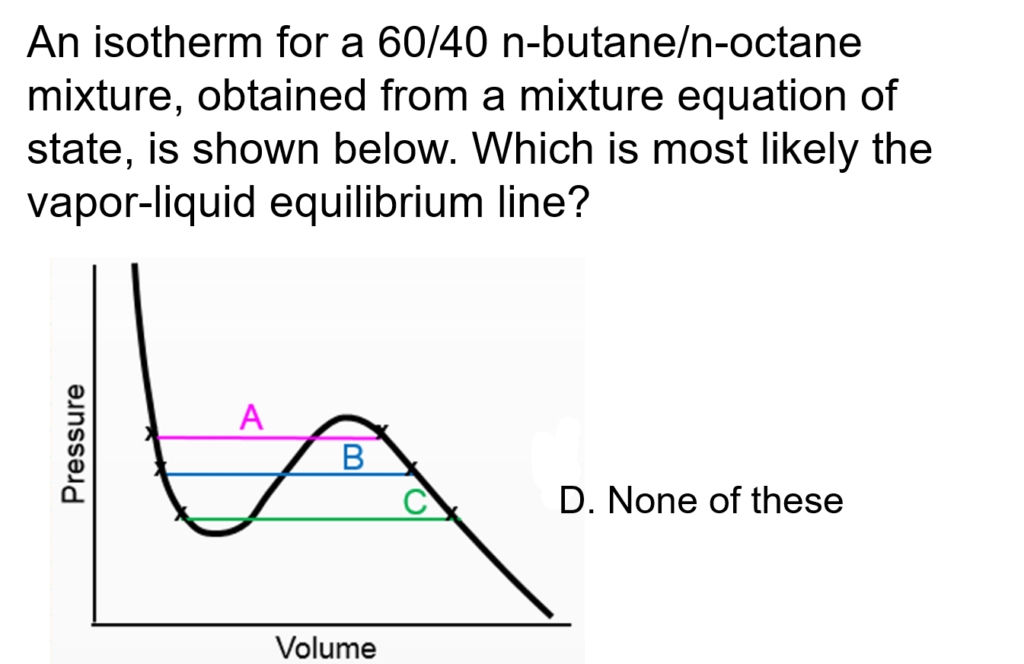LearnChemE

#### Using a Cubic EOS to Determine Vapor-Liquid Equilibrium: ConcepTest and Example Problem

Try to answer this ConcepTest and solve the example problem before using this module. Studies show that trying to answer the questions before studying material improves learning and retention. We suggest that you write down the reasons for your answers. By the end of this module, you should be able to answer these on your own. Answers will be given at the end of this module.##### Example Problem 1

Download the spreadsheet (spreadsheet name: PRFUG) for the Peng-Robinson equation of state for mixtures to determine vapor-liquid equilibrium. Determine the fugacity coefficients in both liquid and vapor phases to calculate the bubble point pressure and vapor composition. Given below are the temperature and liquid compositions. Determine the vapor compositions, yi and the pressure.
xpentene = 0.20    xhexane = 0.30    xheptane = 0.50     T = 315 K